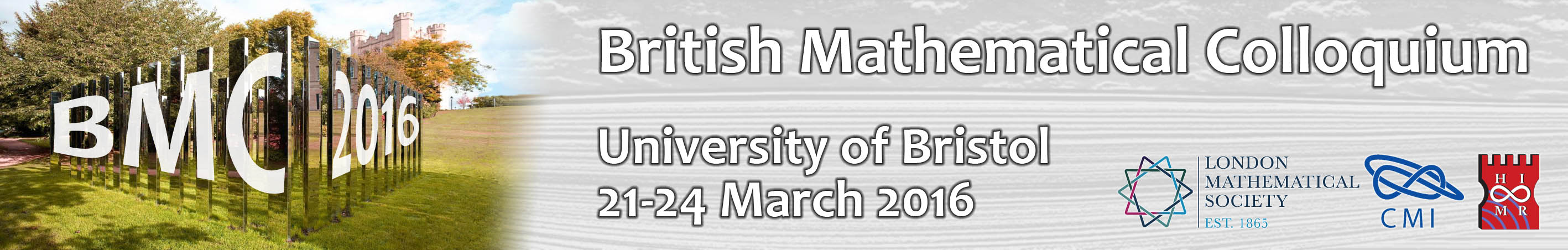# Speed talks

## Wednesday 17:00-18:00

### LT1: Combinatorics, Topology, Geometry, Probability, Dynamical systems - chaired by Ben Barber

Vasso Anagnostopoulou (Imperial College London)
A model for the non-autonomous Hopf bifurcation [Dynamical Systems]

Benjamin Bode (University of Bristol)
Knots and Singularities [Geometric Topology]

Alexander Giles (Bristol Univeristy)
Random Walks on Random Geometric Graphs [Random Graphs and Lattices]

Istvan Kolossvary (Alfred Renyi Institute of Mathematics)
Small World Phenomena for Random Apollonian Networks [Probability]

Jason Semeraro (University of Bristol)
Using tournaments to estimate Turan densities for hypergraphs [Combinatorics]

The Erdos-Rothschild problem on edge-colourings [Combinatorics]

### LT2: Number Theory, Ergodic Theory, Harmonic Analysis - chaired by Kevin Hughes

Jolanta Marzec (University of Bristol)
Hecke eigenvalues vs Fourier coefficients [Number Theory]

Dan Fretwell (Heilbronn Institute)
Towards a "convoluted" problem of Cohn [Harmonic Analysis/Number Theory]

Lee Butler (University of Bristol)
Proving that Apery's constant is irrational in five minutes [Number theory]

Jimmy Tseng (University of Bristol)
Counting integral solutions in thinning regions using the Birkhoff ergodic theorem [Ergodic theory and number theory]

Bruno Brogni Uggioni (Warwick University)
Convolution of Measures on the Symbolic Space [Ergodic Theory]

Andrew Corbett (University of Bristol)
The four-norm of a modular form [Number theory]

Samuele Anni (Warwick University)
Congruence graphs [Number theory]

David Spencer (University of Sheffield)
Congruences of local origin [Number Theory]

Thomas Oliver (University of Bristol)
Low Degree L-Data [Number Theory]